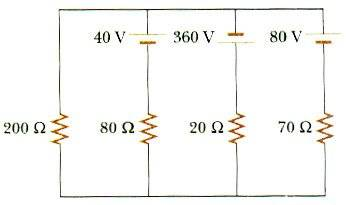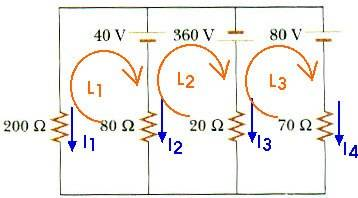# Kirchoff's Rules- Problem

## Homework Statement

In the circuit of Figure P28.28, determine the current in each resistor and the potential difference across the 200-ohm resistor.Kirchoff's Rules
V=IR

## The Attempt at a Solution

The way I did it was like this:I set up a system of equations-
I1 + I2 + I3 + I4 = 0
- 40 V = I2 (80Ω) - I1 (200Ω)
40 V - 360 V = I3 (20Ω) - I2 (80Ω)
- 360 V - 80 V = I4 (70Ω) - I3 (20Ω)

And I get
I1=1.83 (for 200Ω)
I2=4.07 (for 80Ω)
I3=.30 (for 20Ω)
I4=-6.2 (for 70Ω)
Then I do V=IR to get the potential difference, which I got was 366 volts.

But in the back of the book, it says the answers are:
1.00 A up in 200Ω
4.00 A up in 70 Ω
3.00 A up in 80 Ω
8.00 A down in 20 Ω
200 V

What did I do wrong